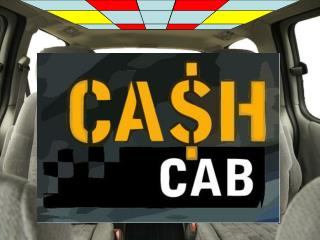DownloadDownload PresentationSTRIKES

# STRIKES

Télécharger la présentation## STRIKES

- - - - - - - - - - - - - - - - - - - - - - - - - - - E N D - - - - - - - - - - - - - - - - - - - - - - - - - - -
##### Presentation Transcript

1. Question 1: Write each improper fraction as a mixed number 17/5 99/10 22/7 STRIKES Answer: 3 2/5, 9 9/10, 3 1/7

2. Question 2: Write each fraction as a decimal ¾ 2/5 9/10 STRIKES Answer: 0.75, 0.4, 0.9

3. Question 2: Write each fraction as a decimal 8/10 2/4 STRIKES X Answer: 0.8, 0.5

4. Question 3: Order from least to greatest ¾, ½, 1/4 STRIKES Answer: ¼, ½, 3/4

5. Question 3:Order from least to greatest ¾, ½, 1/4 STRIKES X Answer: ¼, ½ , 3/4

6. Question 3: 3:Order from least to greatest ¾, ½, 1/4 STRIKES XX Answer: ¼, ½ , 3/4

7. Question 4: Tell if the number is divisible by 2,3,5,10 50 STRIKES Answer: 2, 5, 10

8. Question 4: Tell if the number is divisible by 2,3,5,10 50 STRIKES X Answer: 2, 5, 10

9. Question 4: Tell if the number is divisible by 2,3,5,10 50 STRIKES XX Answer: 2, 5, 10

10. Question 5: Find the GCF 16, 32 STRIKES Answer: 16

11. Question 5: Find the GCF 16, 32 STRIKES X Answer: 16

12. Question 5: Find the GCF 16, 32 STRIKES XX Answer: 16

13. Question 6: Find the GCF 25, 100 STRIKES Answer: 25

14. Question 6: Find the GCF 25, 100 STRIKES X Answer: 25

15. Question 6: Find the GCF 25, 100 STRIKES XX Answer: 25

16. Question 7: Find the LCM 5, 14 STRIKES Answer: 70

17. Question 7: Find the LCM 5, 14 STRIKES X Answer:

18. Question 7: Find the LCM 5, 14 STRIKES XX Answer: 70

19. Question 8: Find the LCM 17, 3 STRIKES Answer: 51

20. Question 8: Find the LCM 17, 3 STRIKES X Answer: 51

21. Question 8: Find the LCM 17, 3 STRIKES XX Answer: 51

22. Question 9: Estimate 12.33 + 3.88 STRIKES Answer: 12 + 4 = 16

23. Question 9: 12.33 + 3.88 STRIKES X Answer: 12 + 4 = 16

24. Question 9: 12.33 + 3.88 STRIKES XX Answer: 12 + 4 = 16

25. Question 10: Estimate 1.009 x 3. 67 STRIKES Answer: 1 x 4 = 4

26. Question 10: Estimate 1.009 x 3. 67 STRIKES X Answer: 1 x 4 = 4

27. Question 10: Estimate 1.009 x 3. 67 STRIKES XX Answer: 1 x 4 = 4

28. Question 11: Find the quotient. Round to the nearest hundredth. \$27.50/3 STRIKES Answer: \$9.17

29. Question 11: Find the quotient. Round to the nearest hundredth. \$27.50/3 STRIKES X Answer: \$9.17

30. Question 11: Find the quotient. Round to the nearest hundredth. \$27.50/3 STRIKES XX Answer: \$9.17

31. Question 12: Find the quotient. Round to the nearest hundredth. \$152.30/3 STRIKES Answer: \$50.77

32. Question 12: Find the quotient. Round to the nearest hundredth. \$152.30/3 STRIKES X Answer: \$50.77

33. Question 12: Find the quotient. Round to the nearest hundredth. \$152.30/3 STRIKES XX Answer: \$50.77

34. Get out of my cab! SCORE STRIKES \$ 0 XXX